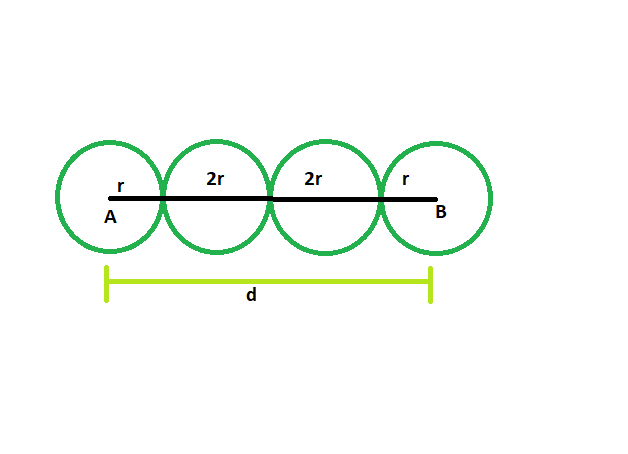# Find the radius of the circles which are lined in a row, and distance between the centers of first and last circle is given

• Last Updated : 31 May, 2022

Given here are n circles which touch each other externally, and are lined up in a row. The distance between the centers of the first and last circle is given. The circles have a radius of equal length. The task is to find the radius of each circle.
Examples:

```Input: d = 42, n = 4
Output: The radius of each circle is 7

Input: d = 64, n = 5
Output: The radius of each circle is 8```Approach
Suppose there are n circles each having radius of length r
Let, the distance between the first and last circles = d
From the figure, it is clear,
r + r + (n-2)*2r = d
2r + 2nr – 4r = d
2nr – 2r = d
so, r = d/(2n-2)## C++

 `// C++ program to find radii of the circles` `// which are lined in a row` `// and distance between the` `// centers of first and last circle is given`   `#include ` `using` `namespace` `std;`   `void` `radius(``int` `n, ``int` `d)` `{` `    ``cout << ``"The radius of each circle is "` `         ``<< d / (2 * n - 2) << endl;` `}`   `// Driver code` `int` `main()` `{` `    ``int` `d = 42, n = 4;` `    ``radius(n, d);` `    ``return` `0;` `}`

## Java

 `// Java program to find radii of the circles` `// which are lined in a row` `// and distance between the` `// centers of first and last circle is given` `import` `java.io.*;`   `class` `GFG` `{`   `static` `void` `radius(``int` `n, ``int` `d)` `{` `    ``System.out.print( ``"The radius of each circle is "` `        ``+d / (``2` `* n - ``2``));` `}`   `// Driver code` `static` `public` `void` `main (String []args)` `{` `    ``int` `d = ``42``, n = ``4``;` `    ``radius(n, d);` `}` `}`   `// This code is contributed by anuj_67..`

## Python3

 `# Python program to find radii of the circles` `# which are lined in a row` `# and distance between the` `# centers of first and last circle is given`   `def` `radius(n, d):` `    ``print``(``"The radius of each circle is "``,` `        ``d ``/` `(``2` `*` `n ``-` `2``));`   `d ``=` `42``; n ``=` `4``;` `radius(n, d);`     `# This code is contributed by PrinciRaj1992`

## C#

 `// C# program to find radii of the circles` `// which are lined in a row` `// and distance between the` `// centers of first and last circle is given` `using` `System;`   `class` `GFG` `{`   `static` `void` `radius(``int` `n, ``int` `d)` `{` `    ``Console.Write( ``"The radius of each circle is "` `        ``+d / (2 * n - 2));` `}`   `// Driver code` `static` `public` `void` `Main ()` `{` `    ``int` `d = 42, n = 4;` `    ``radius(n, d);` `}` `}`   `// This code is contributed by anuj_67..`

## Javascript

 ``

Output:

`The radius of each circle is 7`

Time Complexity: O(1)

Auxiliary Space: O(1)

My Personal Notes arrow_drop_up
Recommended Articles
Page :